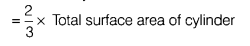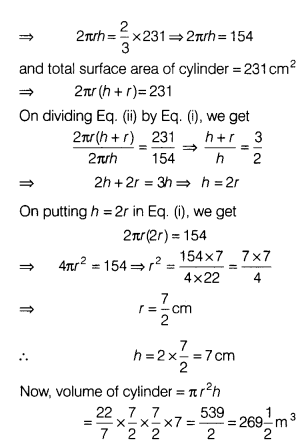# A solid cylinder has a total surface area of 231m2. Its curved surface area is 2/3 of the total

A solid cylinder has a total surface area of 231m2. Its curved surface area is 2/3 of the total surface area. Then, find the volume of the cylinder.

Given, total surface area of cylinder = 231 m2 Let the radius and height of cylinder are r and h. According to the question,
Curved surface area of cylinder